> with(linalg):

> M:= matrix([[1, 1,1],[0, 1, 0], [-1, -1, 1]]);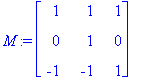> det(M);#This gives back the determinant of M.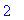> adj(M);#This gives back the adjoing matrix adj(M) of M.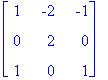> multiply(M, adj(M));#A matrix M multiplied by its adjoint gives a diagonal matrix whose diagonal elements are all equal to det(M).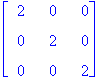> # Here are some more basic operations:

> # the transpose of M is found by:

> transpose(M);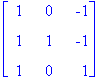> #The ij minor (the whole matrix obtained by deleting the i-th row and j-th column; we sometimes use the term minor for the determinant of this matrix) is found by:

> minor(M, 2, 1);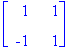> minor(M, 1, 1);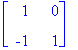> # Here are some examples of special matrices, called hilbert matrices.

> for i from 1 to 4 do print(hilbert(i)) od;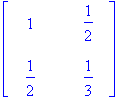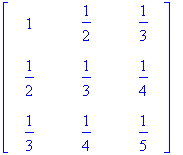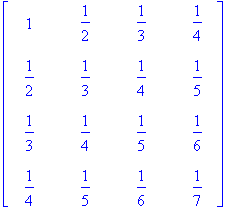> #We would like to find the determinant of Hilbert(n), if there is a nice formula....

> for i from 1 to 5 do print(det(hilbert(i))) od;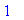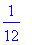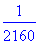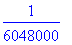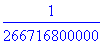> # Can you find the formula?!

> #The vandermonde matrix is given by:

> vandermonde([x, y, z]);> # and its determinant is:

> det(vandermonde([x, y, z]));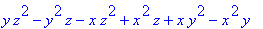> #We may factor the last result refering to it as %:

> factor(%);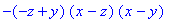> # You may also put numbers into the vandermonde:

> vandermonde([1, 2, 3, 4, 5]);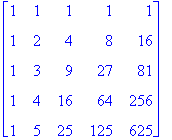>